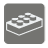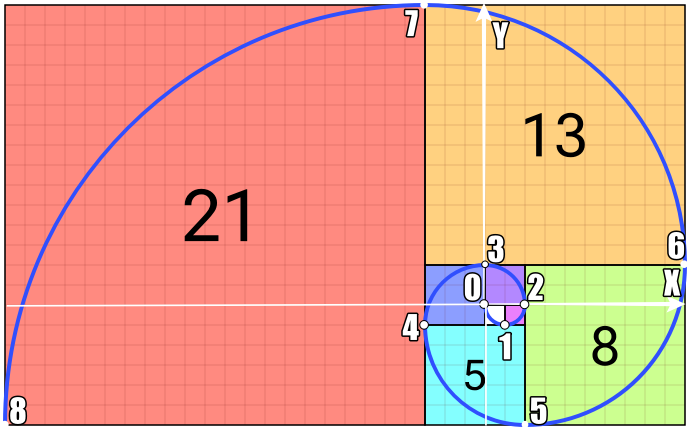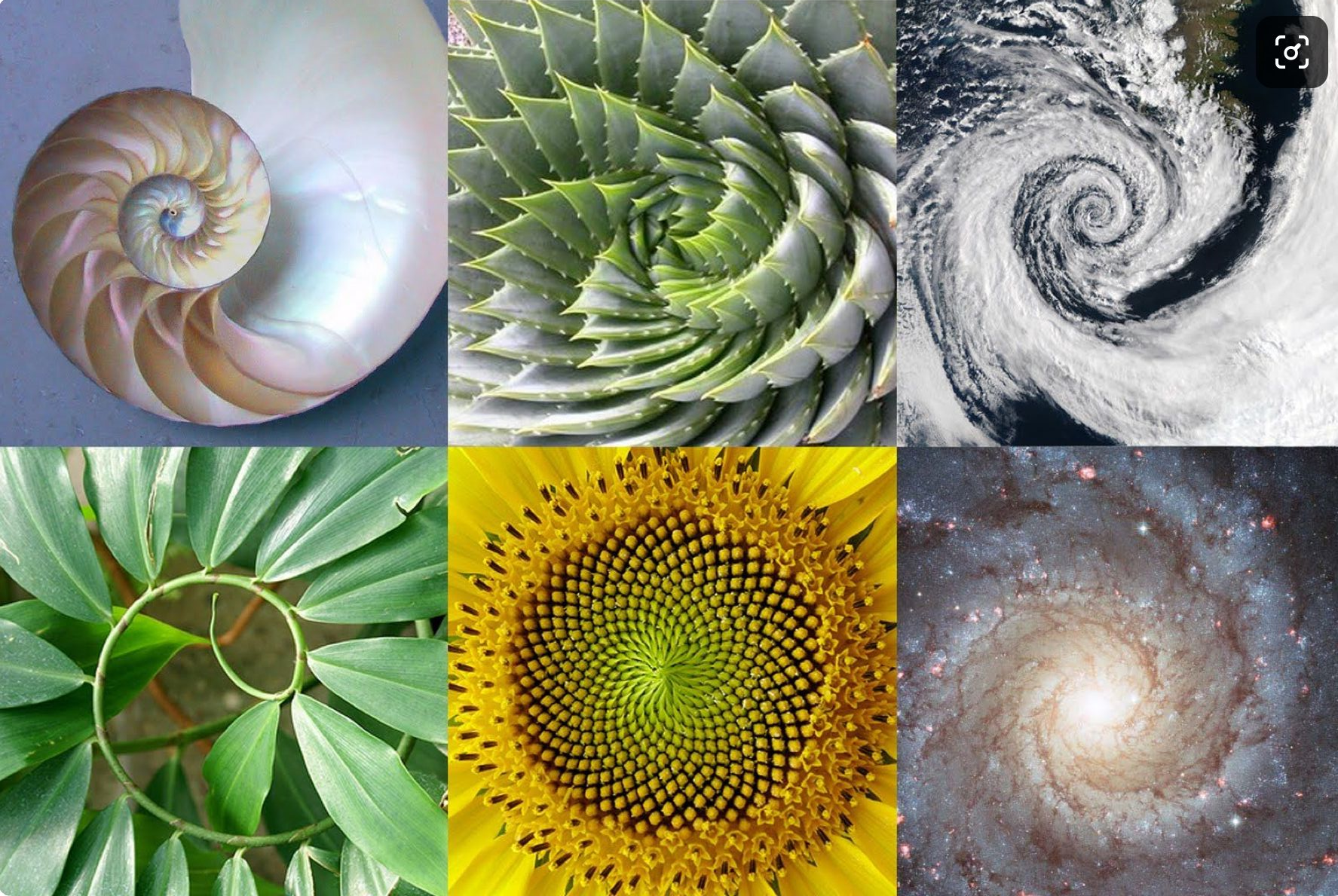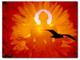# Fibonacci Spiral's End

Undefined
English UK

I believe, you have heard about Fibonacci numbers, which form a respective sequence, where each number is the sum of the two preceding ones. The sequence commonly starts from 0 and 1:

`0, 1, 1, 2, 3, 5, 8, 13, 21, 34, 55, 89, 144, ...`
 The sequence may be shown as a tiling with squares whose side lengths are successive Fibonacci numbers. For this mission we use exactly this position of squares: If you follow numbers, you see that they form a spiral. Indeed, on the next image the Fibonacci spiral is build: an approximation of the golden spiral, created by drawing circular arcs, connecting the opposite corners of squares in the Fibonacci tiling:

Now let's put x-axis and y-axis on the image with 0 point in the start of the spiral. Each numbered dot marked is the corner of the square (or the very start of the spiral) of i-th number, where the spiral ends if i-th number is the last one:So, for the given sequence number, your function should return the coordinates [x, y] of the end of the spiral (square corner) for the respective Fibonacci number. let's look at the table:

 Sequence number (function input) Respective Fibonacci number Coordinates of the corner (spiral's end) 0 1 2 3 4 5 6 7 8 ... 0 1 1 2 3 5 8 13 21 ... [0, 0] [1, -1] [2, 0] [0, 2] [-3, -1] [2, -6] [10, 2] [-3, 15] [-24, -6] ...

Input: Integer.

Output: List of two integers.

Examples:

```assert fibo_spiral_end(0) == [0, 0]
assert fibo_spiral_end(1) == [1, -1]
assert fibo_spiral_end(2) == [2, 0]
assert fibo_spiral_end(3) == [0, 2]
```How it’s used: Fibonacci numbers appear unexpectedly often in mathematics, so much so that there is an entire journal dedicated to their study, the Fibonacci Quarterly. Applications of Fibonacci numbers include computer algorithms such as the Fibonacci search technique and the Fibonacci heap data structure, and graphs called Fibonacci cubes used for interconnecting parallel and distributed systems. They also appear in biological settings, such as branching in trees, the arrangement of leaves on a stem, the fruit sprouts of a pineapple, the flowering of an artichoke, an uncurling fern, and the arrangement of a pine cone's bracts.37
Settings
Code:
Other:
Invalid hot key. Each hot key should be unique and valid
Hot keys:
•  to Run Code: to Check Solution: to Stop:
CheckiO Extensions

CheckiO Extensions allow you to use local files to solve missions. More info in a blog post.

In order to install CheckiO client you'll need installed Python (version at least 3.8)

Install CheckiO Client first:

`pip3 install checkio_client`

`checkio --domain=py config --key=`

Sync solutions into your local folder

`checkio sync`

(in beta testing) Launch local server so your browser can use it and sync solution between local file end extension on the fly. (doesn't work for safari)

`checkio serv -d`

Alternatevly, you can install Chrome extension or FF addon

`checkio install-plugin`
`checkio install-plugin --ff`
`checkio install-plugin --chromium`

Read more here about other functionality that the checkio client provides. Feel free to submit an issue in case of any difficulties.